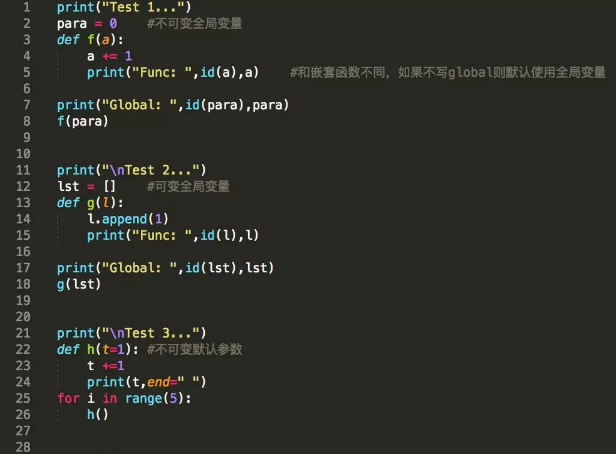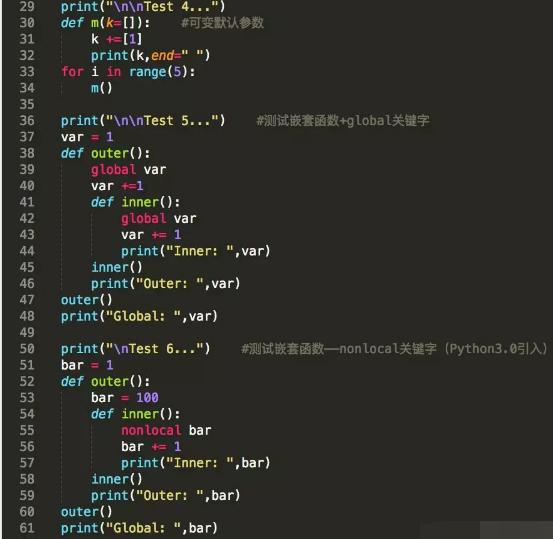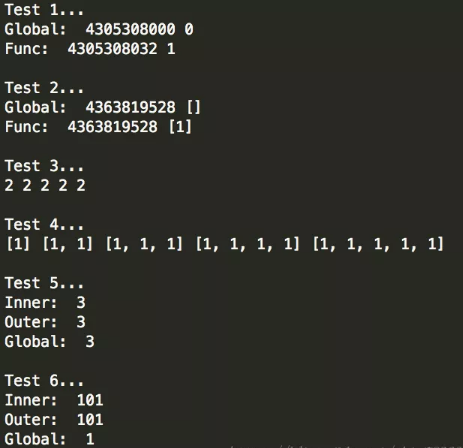## Python传入参数的几种方法 原

Python唯一支持的参数传递方式是『共享传参』（call by sharing）

``````在学习过程中有什么不懂得可以加我的
python学习扣扣qun，784758214

def func(a, b):
print(a+b)
func(1, 2)  #3
``````

``````def power(x, n):
s = 1
while(n > 0):
n -= 1
s *= n
return s
power(2, 3) #8
``````

``````def power(x, n=2):
s = 1
while(n > 0):
n -= 1
s *= n
reutrn s
power(3)    #9
power(2, 3) #8
``````

``````#以下这个函数如果被多次调用会在默认添加多个END字符串
l.append('END')
return l
#为了避免这个问题，应该把传入的默认参数设置为不可变的
l = []
l.append('END')
return l
``````

``````#传入一个列表，严格地说这不是可变参数
def calc(l):
sum = 0
for n in l:
sum += n
return sum
>>> calc([1,2,3])
7
#这才是可变参数，虽然在使用上和列表没有区别，但是参数nums接收到的是一个tuple（这些参数在传入时被自动组组装为一个元祖）
def calc(*nums):
sum = 0
for n in nums:
sum += n
return sum
>>> calc(1,2,3)
7
>>> my_ls = [1,2,3]
>>> calc(*my_ls)
7
``````

``````在学习过程中有什么不懂得可以加我的
python学习扣扣qun，784758214

#用两个星号表示关键字参数
def person_info(name, age, **kw):
print("name", name, "age", age, "other", kw)
>>> person_info("Xiaoming", 12)
name Xiaoming age 12 other{}
>>> person_info("Dahuang", 35, city = "Beijing")
name Dahuang age 35 other {'city':'Beijing'}
``````

``````#这里星号分割符后面的city、job是命名关键字参数
person_info(name, age, *, city, job):
print(name, age, city, job)
>>> person_info("Alex", 17, city = "Beijing", job = "Engineer")
Alex 17 Beijing Engineer    #看来这里不再被自动组装为字典
``````

``````#Python学习交流QQ群：857662006
#args是变长参数，而city和job是命名关键字参数
person_info(name, age, *args, city, job):
print(name, age, args, city)
>>> person_info("Liqiang", 43, "balabala", city = "Wuhan", job = "Coder")
Liqiang 43 balabala Wuhan Coder
``````

``````def f1(a, b, c=0, *args, **kw):
print("a = ", a, "b = ", b, "args = ", args, "kw = ",kw)
def f2(a, b, c=0, *, d, **kw):
print("a = ", a, "b = ", b, "c = ", c, "d = ", d, "kw = ", kw)
>>> f1(1, 2)
a = 1 b = 2 c = 0 args =() kw = {}
>>> f1(1, 2, c=3)
a = 1 b = 2 c = 3 args = () kw = {}
>>> f1(1, 2, 3, 'a', 'b')
a = 1 b = 2 c = 3 args = ('a', 'b') kw = {}
>>> f1(1, 2, 3, 'a', 'b', x = 99)
a = 1 b = 2 c = 3 args = ('a', 'b') kw = {'x':99}
>>> f2(1, 2, d = 99, ext = None)
a = 1 b =2 c = 0 d = 99 kw = {'ext':None}
``````

1）参数的传递是通过自动将对象赋值给本地变量名来实现的 函数参数在实际中只是Python赋值的另一个实例而已，因为引用可以是以指针的形式来实现的，所有的参数实际上都是通过指针进行传递的，作为参数被传递的对象从来不自动拷贝

2）在函数内部的参数名的赋值不会影响调用者 在函数运行时，在函数头部的参数名时一个新的、本地的变量名，这个变量名是在函数的本地作用域内的，函数参数名和调用者作用域中的变量是没有区别的

3）改变函数的可变对象参数的值也许会对调用者有影响 换句话说，因为参数是简单地赋值给传入的对象，函数就能够就地改变传入的可变对象，因此其结果会影响调用者；可变参数对函数来说可以做输入和输出的

Python的通过赋值进行传递的机制与C++的引用参数选项不完全相同，但是实际中，它与C语言的参数传递模型相当类似：

1）不可变参数“通过值”进行传递 像整数和字符串这样的对象是不可变对象，它们通过对象引用而不是拷贝进行传递的，但是因为无论如何都不可能在原处改变不可变对象，实际的效果就很像创建了一份拷贝

2）可变对象是通过“指针”进行传递的 列表和字典这样的对象也是通过对象引用进行传递的，这一点与C语言使用指针传递数组很相似，可变对象能够在函数内部进行原处的改变，这一点和C数组很像### 评论(0)

yzy121403725
2018/06/27
0
0
Python 4 种不同的存取文件骚操作

2018/06/20
0
0
Python里面这些点，据说80%的新手都会一脸懵逼

Python虽然语法简单，通俗易懂，但是再简单它也是一门语言，就像一棵大树，总有一些树枝是弯弯绕绕的，让新手看完之后一脸懵逼，今天我们就来说说这几个点，反正我学的时候是很困惑的！ 01.函...

2018/10/14
59
1
Python自学笔记之函数1——调用函数

twobeings
2015/03/19
130
0

02%
2018/09/02
0
0

0228 我的潘多拉

46分钟前
18
0
OSChina 周六乱弹 —— 屁会不会传染病毒

Osc乱弹歌单（2020）请戳（这里） 【今日歌曲】 @薛定谔的兄弟 ：分享洛神有语创建的歌单「我喜欢的音乐」: 《ハレハレヤ（朗朗晴天）》- 猫瑾 手机党少年们想听歌，请使劲儿戳（这里） @空格...

59分钟前
53
1

27
0

1.大项目工程且多人维护的弊端 （1）项目团队协同成本高，业务响应越来越慢 （2）应用复杂度已超出人的认知负载（向杂乱的电线一样） （3）错误难于隔离（一个模块出错，整个系统挂掉） （4...

zxx901221

68
0
eclipse 上传jar到远程仓库

63
0